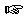### Section 2

Problem 1:
Write the contract, purpose, and header for a function that computes the area of a rectangle given its length and width.

Write three examples for the behavior of this program.Solution

Problem 2:
Develop a function that computes the distance a boat travels across a river, given the width of the river, the boat's speed perpendicular to the river, and the river's speed. Speed is distance/time, and the Pythagorean theorem is c2 = a2 + b2.Solution

Problem 3:
Develop a function that computes how long after their deparature two trains will meet. Assume that the trains travel between two points, along a single section of track, going in opposite directions. The function should consume the trains' speeds and the starting distance between the trains. Speed is distance/time.Solution

Problem 4:
Develop a function that when given an initial amount of money (called the principal), a simple annual interest rate, and a number of months will compute the balance at the end of that time. Assume that no additional deposits or withdrawals are made and and that a month is 1/12 of a year. Total interest is the product of the principal, the annual interest rate expressed as a decimal, and the number of years.Solution

Problem 5:
Develop a function that when given the length and width of a rectangular floor and the edge length of a square tile will compute the whole number of tiles needed to cover the floor completely.Solution

Problem 6:
Develop a function that computes the area of a regular polygon given the length of one side and the number of sides. If n is the number of sides and s is the length of a side, the area of a regular polygon is equal to 1/4 * n * s2 * 1/(tan PI/n).Solution

Problem 7:
Develop a function to return the mean of 5 exam scores.Solution

Problem 8:
Develop a function that when given the area of a square will calculate its perimeter.Solution

Problem 9:
Drop a rubber ball from a height h. Each time it hits the ground, the ball bounces up to 2/3 of the height it dropped. Develop a function that computes how far the ball travels by the time it hits the ground for the third time? Hint: try 36[in] for the initial height.Solution

Problem 10:
contributed by Professor Stephen Bloch, Adelphi University

Develop the function, total-inches. The function consumes a length represented by two numbers: the first a number of feet, and the second a number of inches. The function produces the total length in inches.Solution

Problem 11:
contributed by Professor Stephen Bloch, Adelphi University

The nation of Progressiva has a simple tax code. The tax you pay is your salary times the tax rate, and the tax rate is 1/2% per thousand dollars of salary. For example, if you make \$40,000, your tax rate is 1/2% times 40, which is 20%, so you pay 20% of \$40,000, which is \$8,000.

Develop a function to compute the net pay (i.e. pay after taxes) of a person with a given salary. HINT: develop one or two auxiliary functions as well as `net-pay`.

This tax system has the peculiar feature that, beyond a certain income level, it doesn't pay to earn any more: for every additional dollar you earn, you have to pay MORE than a dollar more to the government. Use your net-pay function to find this income level.

[Note to teachers: this question can be solved easily using differential calculus, but encourage students to find the answer by experiment, even if they've studied differential calculus.]Solution

Problem 12:
contributed by Karen O'Loughlin, Ankeny High School

Develop the function, semester-grade. The function consumes two numbers -- the first representing a cumulative homework score and the other representing the final exam socre -- and produces the semester grade weighted 80% homework and 20% final exam.Solution

Problem 13:
contributed by Karen O'Loughlin, Ankeny High School

Develop the function, winning-average. The function consumes two numbers representing the number of wins and losses and produces the winning average where 1000 would be equivalent to 100 percent.Solution

Problem 14:
contributed by Susan Barocas
1. Write a contract for a function that finds the number of inches in a given number of feet.
2. Write a purpose and contract for a function (operation) that finds the gas consumption (miles per gallon) for a particular vehicle.
3. Write a purpose and contract for a function that computes the slope of a line if the change in y-value and the change in x-value are given.
4. Write the purpose, contract and two examples for a function that consumes the length, width, and depth of a tank and produces its volume.
5. Write the purpose, contract, two examples, and header for a function that consumes five quiz grades for a student and produces the quiz average.
6. Write the purpose, contract, two examples, the header and the body for a function that consumes the amount of money a family spends on groceries per week and that computes the yearly cost of groceries for that family.

Note: This kind of exercise is particularly useful for students who have difficulties with their work habits. It progressively walks them through the very basic design recipe.Solution

Problem 15:
Ms. Sis Y. Fuss wants to push a rock up a hill. The distance is d yards. In one minute, she pushes the rock 30 yards, but then it slides down 4 yards. When she has reached d yards, it won't slide down anymore. How many (entire) minutes will it take her?Solution

 Jamie Raymond Matthias Felleisen 01 december 2003